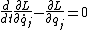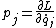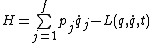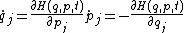# Hamilton's equations of motion

## Hamilton's equations of motion

The motion of a mechanical system may be described by a set of first-order ordinary differential equations known as Hamilton's equations. Because of their remarkably symmetrical form, they are often referred to as the canonical equations of motion of a system. They are equivalent to Lagrange's equations, but the fact that they are of first order and highly symmetrical makes them advantageous for general discussions of the motion of systems. See Lagrange's equations

Hamilton's equations can be derived from Lagrange's equations. Let the coordinates of the system be qj (j = 1, 2, . . . , f), and let the dynamical description of the system be given by the lagrangian L(q, …, t), where q denotes all the coordinates and a dot denotes total time derivative. Lagrange's equations are then given by Eq. (1). The momentum pj canonically conjugate to qj is defined by Eq. (2).

(1)(2)The hamiltonian H is defined by Eq. (3). Then Hamilton's canonical equations are Eqs. (4).

(3)(4)As they stand, Hamilton's equations are no easier to integrate directly than Lagrange's. Hamilton's equations are of great advantage in more general discussions, and they permit the making of canonical transformations which can lead to simplifications. See Canonical transformations

The hamiltonian function H of classical mechanics is used to form the quantum-mechanical hamiltonian operator.

McGraw-Hill Concise Encyclopedia of Physics. © 2002 by The McGraw-Hill Companies, Inc.

## Hamilton's equations of motion

[′ham·əl·tənz i¦kwā·zhənz əv ′mō·shən]
(mechanics)
A set of first-order, highly symmetrical equations describing the motion of a classical dynamical system, namely j = ∂ H /∂ pj , j = -∂ H /∂ qj ; here qj (j = 1, 2,…) are generalized coordinates of the system, pj is the momentum conjugate to qj , and H is the Hamiltonian. Also known as canonical equations of motion.
McGraw-Hill Dictionary of Scientific & Technical Terms, 6E, Copyright © 2003 by The McGraw-Hill Companies, Inc.
Mentioned in ?
References in periodicals archive ?
Having formed the Hamiltonian, the dynamics of the DNA with double helix molecule can be understood by constructing the Hamilton's equations of motion corresponding to the Hamiltonian (1) as
In this section, we will consider the dynamics of two DNA double helix molecules by constructing the Hamilton's equations of motion as
Hamilton's equations of motion (42) can be linearized and subsequently solved approximately by assuming that

Site: Follow: Share:
Open / Close